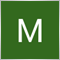16

```extern double  BE_T_1      = 50;
extern double  BE_M_1      = 5;

extern double  BE_T_2      = 100;
extern double  BE_M_2      = 50;

extern double  BE_T_3      = 150;
extern double  BE_M_3      = 100;

double BE_S_M;
double BE_S_T;

void MOVE_BE_1()

{
for(int b=OrdersTotal()-1;b>=0;b--)
{

if(OrderMagicNumber()!=M_Number)continue;
if(OrderSymbol()==Symbol())
if(Bid-OrderOpenPrice()>BE_S_T*Pips)
if(OrderOpenPrice()>OrderStopLoss())
if(!OrderModify(OrderTicket(),OrderOpenPrice(),OrderOpenPrice()+(BE_S_M*Pips),OrderTakeProfit(),0,CLR_NONE))
Print("eror");
}

for(int s=OrdersTotal()-1;s>=0;s--)
{
if(OrderMagicNumber()!=M_Number)continue;
if(OrderSymbol()==Symbol())
if(OrderType()==OP_SELL)
if(OrderOpenPrice()<OrderStopLoss())
if(!OrderModify(OrderTicket(),OrderOpenPrice(),OrderOpenPrice()-(BE_S_M*Pips),OrderTakeProfit(),0,CLR_NONE))
Print("eror");
}

}

```

Hi,

Break even ea is program to move SL from one price to other price, if price pass at certain price. it a nice and straight forward program.

but how to make it trigger more than one time. such as

example current entry price is 1.28000, sl 1.285000 in sell order.

if current price reach 1.175000(50pips), sl move to break even such as to 1.28000(5pips).

EA will not make more modify order after condition are meet.

so how to trigger break even again if price reach 1.17000(100pips), sl move to (1.175000)(50 pips)

and again price reach 1.165000(150pips),sl move to 1.17000(100pips)

i want to make BE_B_M(sl move to(example:5)) and BE_B_T(price reach(example:50)) as variable and every time price reach target variable change to next value

so became BE_B_M(sl move to(example:50)) and BE_B_T(price reach(example:100))